Next: MINIMUM STEINER TREE Up: Spanning Trees Previous: MINIMUM DIAMETER SPANNING SUBGRAPH   Index

### MINIMUM COMMUNICATION COST SPANNING TREE

• INSTANCE: Complete graph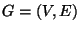, weight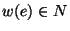for each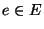, requirement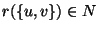for each pair of vertices from V.

• SOLUTION: A spanning tree for G.

• MEASURE: The weighted sum over all pairs of vertices of the cost of the path between the pair in T, i.e.,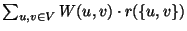, where W(u,v) denotes the sum of the weights of the edges on the path joining u and v in T.

• Good News: Approximable within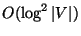if the weight function satisfies the triangle inequality .

• Comment: MINIMUM ROUTING COST SPANNING TREE, the variation in whichfor all u,v, admits a PTAS .

• Garey and Johnson: ND7

Viggo Kann
2000-03-20